# For the below ME alternatives, which machine should be selected based on the AW analysis. MARR=10%

QUESTION 3

For the below ME alternatives, which machine should be selected based on the AW analysis. MARR=10%

Machine AMachine BMachine C
First cost, $26,5383000010000 Annual cost,$/year8,0606,0004,000
Salvage value, $4,0005,0001,000 Life, years362 Answer the below questions: A- AW for machine A= QUESTION 4 For the below ME alternatives, which machine should be selected based on the AW analysis. MARR=10% Machine AMachine BMachine C First cost,$1500021,66710000

Annual cost, $/year8,8706,0004,000 Salvage value,$4,0005,0001,000

Life, years362

B- AW for machine B=

3)

Annual worth = Net present worth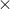Capital recovery factor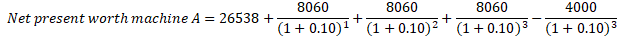Net present worth of machine A = $43576.76784 Annual worth of machine A =$ 43576.76784( A/P, 10%, 3 years)

( A/P, 10%, 3 years) = Capital recovery factor

Annual worth of machine A = $43576.767840.4021 Annual worth of machine A =$ 17522.22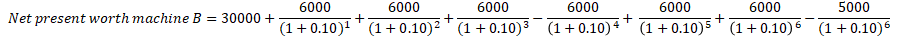Net present worth machine B = $53309.2 Annual worth of machine B =$ 53309.2( A/P, 10%, 6 years)

Annual worth of machine B = $53309.20.2296 Annual worth of machine B =$ 12239.79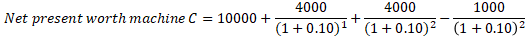Net present worth of machine C = $16115.7 Annual worth of machine C =$ 16115.7( A/P, 10%, 2 years)

Annual worth of machine C =   $16115.70.5762 Annual worth of machine C =$ 9285.87

Based on the annual worth analysis, machine C must be selected because it has the lowest annual worth of costs compared to machine A and machine B.

---------------------------------------------------------------------------------------------------------------

4)

Annual worth = Net present worthCapital recovery factor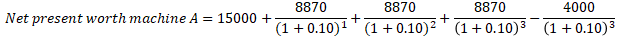Net present worth of machine A = $34053.12 Annual worth =$ 34053.12( A/P, 10%, 3 years)

Annual worth machine A =   $34053.120.4021 =$ 13692.76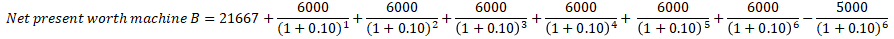Net present worth of machine B = $44976.2 Annual worth machine B =$ 44976.20.2296

Annual worth machine B = $10326.53Annual worth of machine C =$ 16115.7( A/P, 10%, 2 years)

Annual worth of machine C =   $16115.70.5762 Annual worth of machine C =$ 9285.87

Based on the annual worth analysis, machine C must be selected because it has the lowest annual worth of costs compared to machine A and machine B.

SOLUTION :

Q4 :

r = MARR = 10% = 0.1

=> (1 + r) = 1.1

PV of costs of Machine A :

= 26538 + 8060/ 1.1 + 8060/1.1^2 + 8060/1.1^3 - 4000/1.1^3

= 43576.77 ($) PV of costs of Machine B : = 30000 + 6000 (1.1^6 - 1)/(0.1*1.1^6) - 5000/1.1^6 = 53309.19 ($)

PV of costs of Machine C :

= 10000 + 4000/1.1 + 4000/1.1^2 - 1000/1.1^2

= 16115.70 ($) Therefore, as per PV of costs , Machine C has lowest cost. So Machine C should be selected. (ANSWER) Q3 : r = MARR = 10% = 0.1 => (1 + r) = 1.1 PV of costs of Machine A : = 15000 + 8870/ 1.1 + 8870/1.1^2 + 8870/1.1^3 - 4000/1.1^3 = 34053.12 ($)

PV of costs of Machine B :

= 21667 + 6000 (1.1^6 - 1)/(0.1*1.1^6) - 5000/1.1^6

= 44976.19 ($) PV of costs of Machine C : = 10000 + 4000/1.1 + 4000/1.1^2 - 1000/1.1^2 = 16115.70 ($)

Therefore, as per PV of costs , Machine C has lowest cost. So Machine C should be selected.

#### Earn Coin

Coins can be redeemed for fabulous gifts.

Similar Homework Help Questions